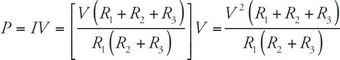# AP Physics 1 Question 89: Answer and Explanation

### Test Information

Question: 89

9.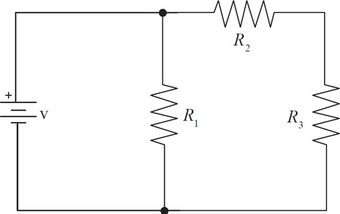Determine the total power dissipated through the circuit shown above in terms of v, R1, R2, and R3.

• A.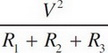• B.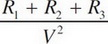• C.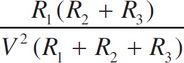• D.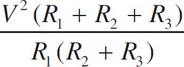Explanation:

D First, we will need to replace all the resistors with an equivalent resistor.

R2 and R3 are in series, so R2 and 3 = R2 + R3.

R1 and R2 and 3 are in parallel, so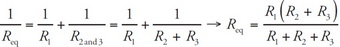.

Second, we need to calculate the current coming out of the battery,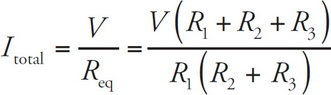Finally, we calculate the power,